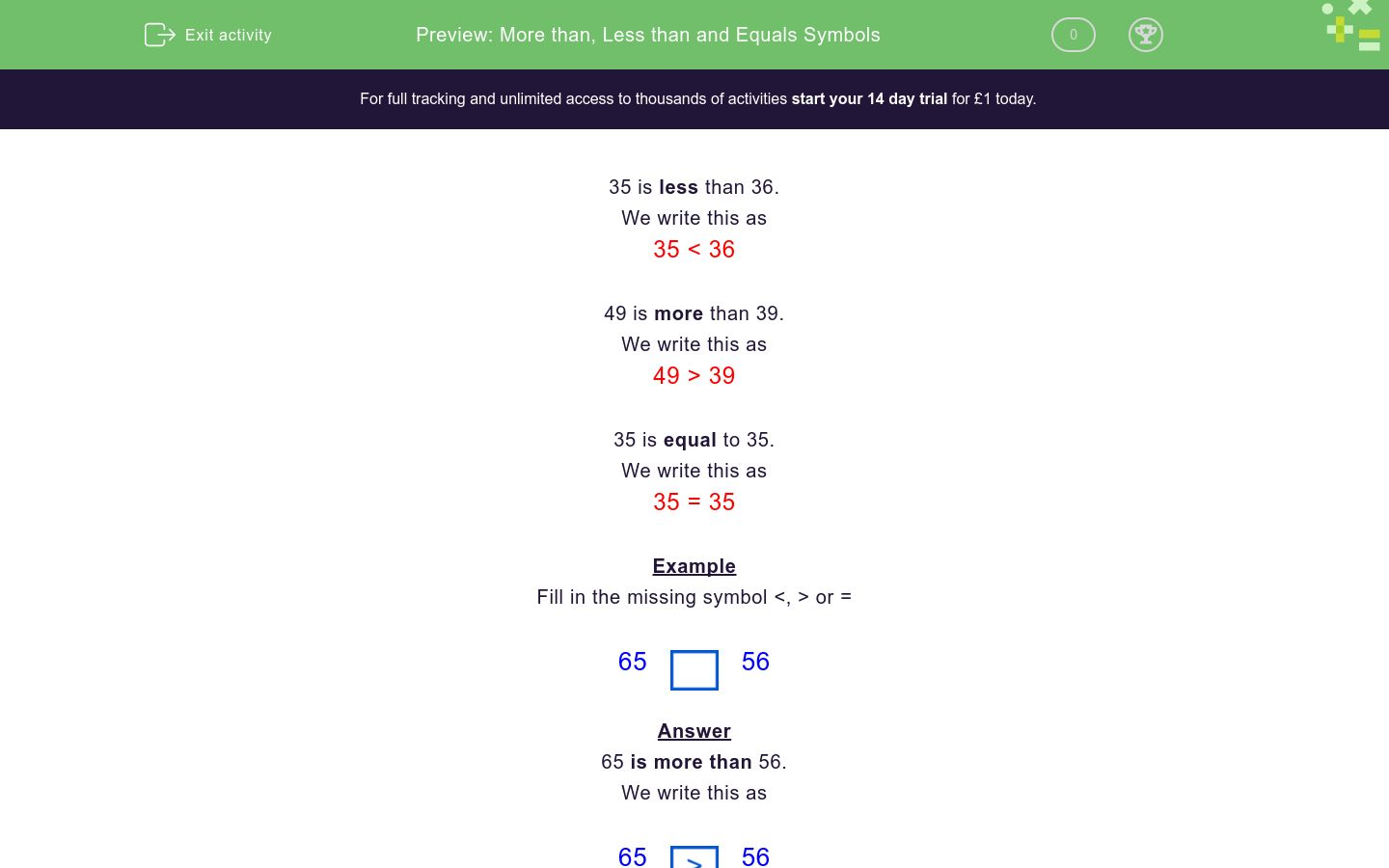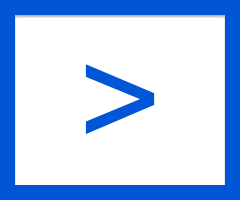# More than, Less than and Equals Symbols

In this worksheet, students must select the >, < or = symbol to enter between two given numbers.Key stage:  KS 1

Curriculum topic:

Curriculum subtopic:   Order/Compare Numbers to 100

Difficulty level:### QUESTION 1 of 10

35 is less than 36.

We write this as

35 < 36

49 is more than 39.

We write this as

49 > 39

35 is equal to 35.

We write this as

35 = 35

Example

Fill in the missing symbol <, > or =

6556

65 is more than 56.

We write this as

6556

Fill in the missing symbol  <,  >  or  =

1516

<

>

=

Fill in the missing symbol  <,  >  or  =

1916

<

>

=

Fill in the missing symbol  <,  >  or  =

4936

<

>

=

Fill in the missing symbol  <,  >  or  =

6968

<

>

=

Fill in the missing symbol  <,  >  or  =

4747

<

>

=

Fill in the missing symbol  <,  >  or  =

7576

<

>

=

Fill in the missing symbol  <,  >  or  =

9999

<

>

=

Fill in the missing symbol  <,  >  or  =

525

<

>

=

Fill in the missing symbol  <,  >  or  =

6556

<

>

=

Fill in the missing symbol  <,  >  or  =

3443

<

>

=

• Question 1

Fill in the missing symbol  <,  >  or  =

1516

<
EDDIE SAYS
15 is less than 16.
• Question 2

Fill in the missing symbol  <,  >  or  =

1916

>
EDDIE SAYS
19 is more than 16.
• Question 3

Fill in the missing symbol  <,  >  or  =

4936

>
EDDIE SAYS
49 is more than 36.
• Question 4

Fill in the missing symbol  <,  >  or  =

6968

>
EDDIE SAYS
69 is more than 68.
• Question 5

Fill in the missing symbol  <,  >  or  =

4747

=
EDDIE SAYS
47 is equal to 47.
• Question 6

Fill in the missing symbol  <,  >  or  =

7576

<
EDDIE SAYS
75 is less than 76.
• Question 7

Fill in the missing symbol  <,  >  or  =

9999

=
EDDIE SAYS
99 is equal to 99.
• Question 8

Fill in the missing symbol  <,  >  or  =

525

<
EDDIE SAYS
5 is less than 25.
• Question 9

Fill in the missing symbol  <,  >  or  =

6556

>
EDDIE SAYS
65 is more than 56.
• Question 10

Fill in the missing symbol  <,  >  or  =

3443

<
EDDIE SAYS
34 is less than 43.
---- OR ----

Sign up for a £1 trial so you can track and measure your child's progress on this activity.

### What is EdPlace?

We're your National Curriculum aligned online education content provider helping each child succeed in English, maths and science from year 1 to GCSE. With an EdPlace account you’ll be able to track and measure progress, helping each child achieve their best. We build confidence and attainment by personalising each child’s learning at a level that suits them.

Get started